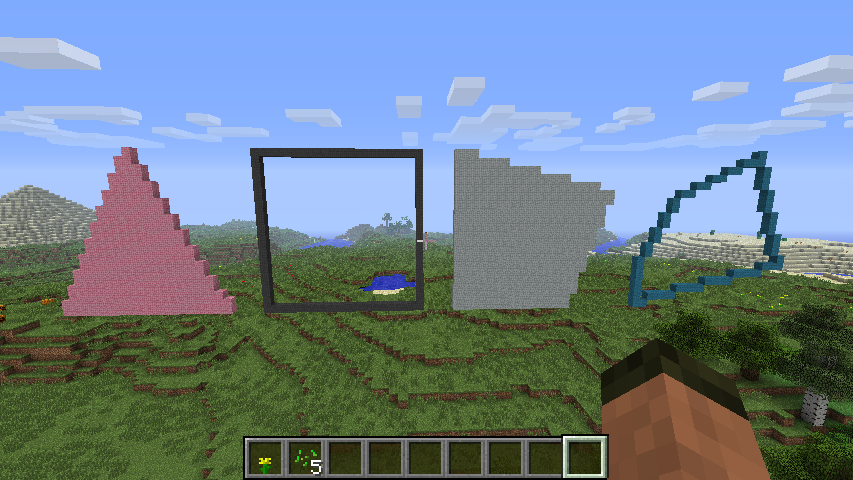# MinecraftDrawing API¶

The MinecraftDrawing API can be used to create lines, circles, spheres, and faces.Example:

```from mcpi.minecraft import Minecraft
from mcpi import block
from minecraftstuff import MinecraftDrawing

mc = Minecraft.create()

mcdraw = MinecraftDrawing(mc)

# draw a diagonal line
mcdraw.drawLine(0,0,0,10,10,10,block.STONE.id)
```

## MinecraftDrawing¶

class `minecraftstuff.``MinecraftDrawing`(mc)

MinecraftDrawing - a class of useful drawing functions

Parameters: mc (mcpi.minecraft.Minecraft) – A Minecraft object which is connected to a world.
`drawCircle`(x0, y0, z, radius, blockType, blockData=0)

draws a circle in the Y plane (i.e. vertically)

Parameters: x0 (int) – The x position of the centre of the circle. y0 (int) – The y position of the centre of the circle. z (int) – The z position of the centre of the circle. radius (int) – The radius of the sphere. blockType (int) – The block id. blockData (int) – The block data value, defaults to `0`.
`drawFace`(vertices, filled, blockType, blockData=0)

draws a face, when passed a collection of vertices which make up a polyhedron

Parameters: vertices (list) – The a list of points, passed as either a `minecraftstuff.Points` object or as a list of `mcpi.minecraft.Vec3` objects. filled (boolean) – If `True` fills the face with blocks. blockType (int) – The block id. blockData (int) – The block data value, defaults to `0`.
`drawHollowSphere`(x1, y1, z1, radius, blockType, blockData=0)

draws a hollow sphere around a point to a radius, sphere has to big enough to be hollow!

Parameters: x1 (int) – The x position of the centre of the sphere. y1 (int) – The y position of the centre of the sphere. z1 (int) – The z position of the centre of the sphere. radius (int) – The radius of the sphere. blockType (int) – The block id. blockData (int) – The block data value, defaults to `0`.
`drawHorizontalCircle`(x0, y, z0, radius, blockType, blockData=0)

draws a circle in the X plane (i.e. horizontally)

Parameters: x0 (int) – The x position of the centre of the circle. y (int) – The y position of the centre of the circle. z0 (int) – The z position of the centre of the circle. radius (int) – The radius of the circle. blockType (int) – The block id. blockData (int) – The block data value, defaults to `0`.
`drawLine`(x1, y1, z1, x2, y2, z2, blockType, blockData=0)

draws a line between 2 points

Parameters: x1 (int) – The x position of the first point. y1 (int) – The y position of the first point. z1 (int) – The z position of the first point. x2 (int) – The x position of the second point. y2 (int) – The y position of the second point. z2 (int) – The z position of the second point. blockType (int) – The block id. blockData (int) – The block data value, defaults to `0`.
`drawPoint3d`(x, y, z, blockType, blockData=0)

draws a single point in Minecraft, i.e. 1 block

Parameters: x (int) – The x position. y (int) – The y position. z (int) – The z position. blockType (int) – The block id. blockData (int) – The block data value, defaults to `0`.
`drawSphere`(x1, y1, z1, radius, blockType, blockData=0)

draws a sphere around a point to a radius

Parameters: x1 (int) – The x position of the centre of the sphere. y1 (int) – The y position of the centre of the sphere. z1 (int) – The z position of the centre of the sphere. radius (int) – The radius of the sphere. blockType (int) – The block id. blockData (int) – The block data value, defaults to `0`.
`drawVertices`(vertices, blockType, blockData=0)

draws all the points in a collection of vertices with a block

Parameters: vertices (list) – A list of `mcpi.minecraft.Vec3` objects. blockType (int) – The block id. blockData (int) – The block data value, defaults to `0`.
`getLine`(x1, y1, z1, x2, y2, z2)

Returns all the points which would make up a line between 2 points as a list

3d implementation of bresenham line algorithm

Parameters: x1 (int) – The x position of the first point. y1 (int) – The y position of the first point. z1 (int) – The z position of the first point. x2 (int) – The x position of the second point. y2 (int) – The y position of the second point. z2 (int) – The z position of the second point.

## Points¶

class `minecraftstuff.``Points`

Points - a collection of minecraft positions or Vec3’s. Used when drawing faces `MinecraftDrawing.drawFace()`.

`add`(x, y, z)

add a single position to the list of points.

Parameters: x (int) – The x position. y (int) – The y position. z (int) – The z position.
`getVec3s`()

returns a list of Vec3 positions